# Rectangle - 4th grade - math problems

#### Number of problems found: 10

• Rectangular field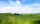What is the area of a rectangular field 100m by 8m?
• Football field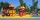The soccer field may have a width of 45 meters. This is 45 meters less than the length of the course. What can be the length of the football field?
• Photo frameA square shape photo with a side length of 20 cm is framed by a 4 cm wide bar. Find the external frame size of this photo (the frame is from all sides)
• A rectangularA rectangular garden 40 m long and 30 m wide is to be fenced with fence posts at each corner. All the other posts will be 5 meters apart. How many posts will be needed to fence in the garden?
• GardenRectangular garden 31 meters 40 centimeters long and 20m30cm wide adjacent with the shorter side with another (fenced) garden and on one longest side is 1 meter wide gate. How many meters of fence is needed to buy? (Estimate, calculate, verify a test calc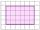Shade the area on the grid that shows 5/8 x 2/4
• Tablecloth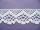The table top is 90 cm long and 50 cm wide. How many cm lace edging is necessary for the tablecloth that hangs on each side by 10 cm?
• Rounding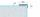What width and length in centimeters may have rectangle land when round the dimensions to the meter, the width is 5 m and length 7 meters?
• RectangleDraw a rectangle with the sides a = 4 cm, b = 5 cm. Mark the center of symmetry S and all axes of symmetry. How many axes of symmetry has? Write down.
• Fence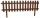How many meters of fencing it is required to purchase if our garden is rectangular measuring 20 m and 180 dm.

We apologize, but in this category are not a lot of examples.
Do you have an exciting math question or word problem that you can't solve? Ask a question or post a math problem, and we can try to solve it.

We will send a solution to your e-mail address. Solved examples are also published here. Please enter the e-mail correctly and check whether you don't have a full mailbox.

Rectangle Problems. Examples for 4th grade.